# Conformal Euclidean space

(diff) ← Older revision | Latest revision (diff) | Newer revision → (diff)

A Riemannian space admitting a conformal mapping onto a Euclidean space. The curvature tensor of a conformal Euclidean space has the form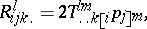(*)

where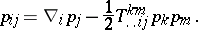For, every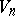is a conformal Euclidean space. In order that a space with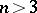be a conformal Euclidean space, it is necessary and sufficient that there exist a tensorsatisfying the conditions (*) and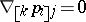. Sometimes a conformal Euclidean space is called a Weyl space admitting a conformal mapping onto a Euclidean space (see ).

How to Cite This Entry:
Conformal Euclidean space. Encyclopedia of Mathematics. URL: http://encyclopediaofmath.org/index.php?title=Conformal_Euclidean_space&oldid=14002
This article was adapted from an original article by G.V. Bushmanova (originator), which appeared in Encyclopedia of Mathematics - ISBN 1402006098. See original article